# Data Science VS Artificial Intelligence VS Machine Learning VS Deep Learning

### Introduction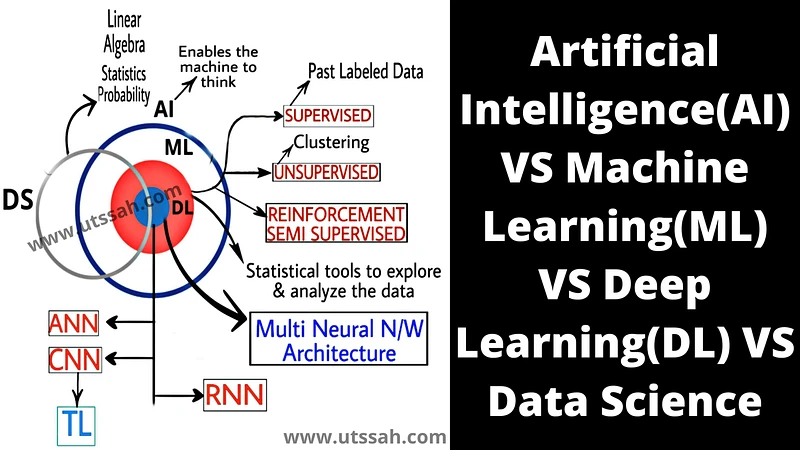SOURCE: www.utssah.com

Data, as the name suggests, is the collection of facts. But, in actuality, it's very different from this definition. Data is something that has no specific meaning. We are surrounded by data like the air and are producing lots of data every second in texts, sounds, images, videos, etc.

Every time we search for any information on Google, Facebook, youtube, etc. The search information is stored in the database to provide us with the best service whenever we revisit those sites and servers. It simply recognizes our interests.

Have you ever wondered how we are handling such a massive amount of data? The task seems to be very complex, and it is.

### Data Science

In simple terms, the science used to process the data to get the information is known as Data Science. Various Machine learning algorithms come into the picture to get the desired results.

### Artificial intelligence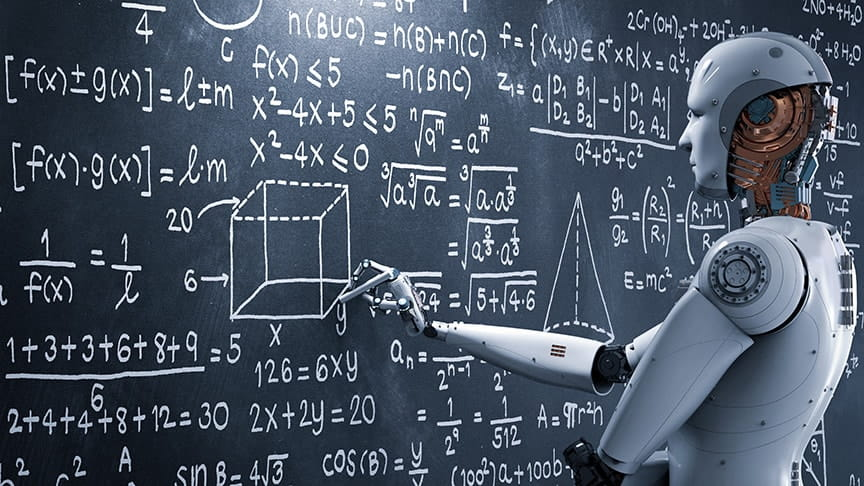Artificial Intelligence enables the machine to think and make decisions without human intervention. We are creating AI-enabled applications like the self-driving car, which could run independently, predicting real-time traffic. AI can revolutionize treatment. Astronomers use AI to find and evaluate exoplanets in distant solar, and from fraud detection to developing new strategies to address climate change. AI uses different mathematical algorithms that make computers understand the relationships between data. The technique sounds similar to Machine Learning.

### Machine Learning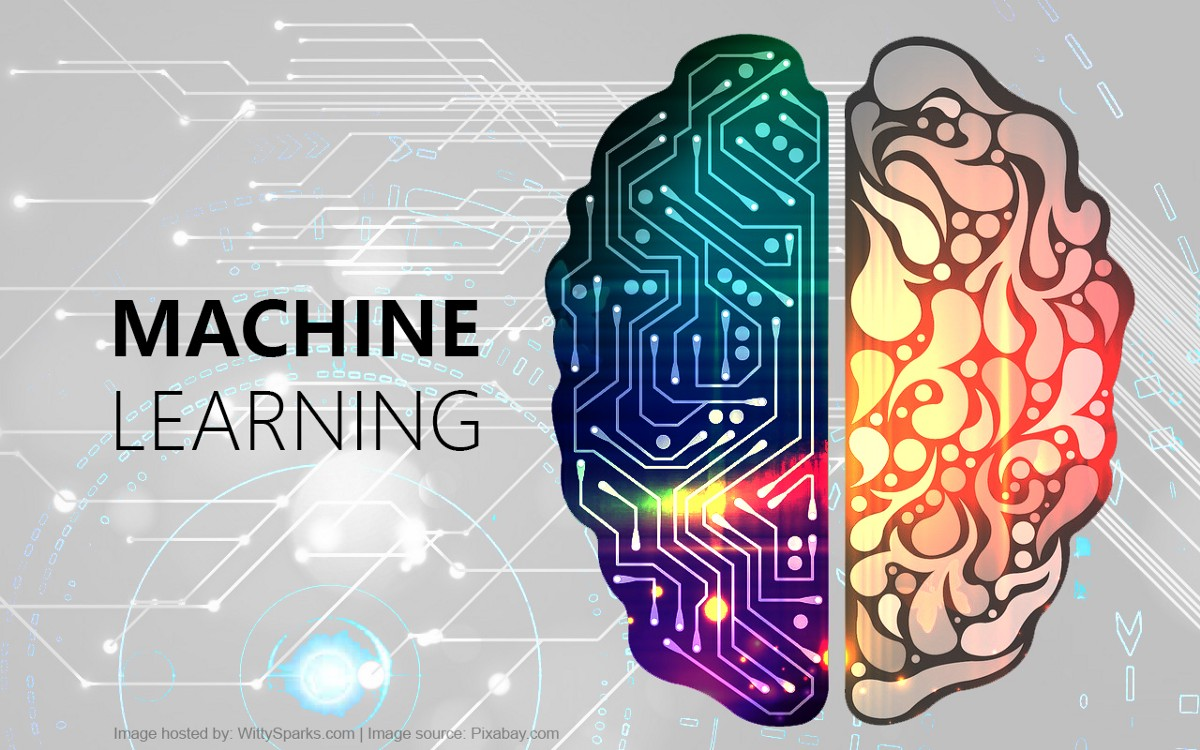SOURCE: towardsdatascience.com

Machine Learning is sometimes used interchangeably with Artificial Intelligence. As a result, the difference between AI and ML can be very unclear.

All Machine Learning algorithms are Artificial Intelligence, but not all Artificial Intelligence is Machine Learning. Machine Learning is a way of achieving Artificial Intelligence; that is, it is the subset of Artificial Intelligence which is a bunch of statistical tools to learn from the data.

Machine Learning is the ability to learn without being explicitly programmed. We can get AI without Machine learning, but that would mean building millions of lines of codes with complex

rules and decisions trees. So instead of heart coding software routines with software instructions to accomplish a particular task, Machine Learning is a way to train an algorithm to learn how. Training involves feeding vast amounts of data to the algorithm, allowing it to adjust itself and improve. Machine learning is of three types.

Supervised Learning, Unsupervised Learning, and Reinforcement Learning. Let's have a brief look at all three.

#### Supervised Learning:

The model is trained with some inputs and outputs from the actual data. i.e., the model first learns the patterns from the information and work of the data and then predicts the outcome for new datasets. Classification and regression problems are common examples. You can go through more details in the link.

#### Unsupervised Learning:

training inputs and outputs do not train the model. It learns on its own, discovering the patterns by classification. The clustering problem is a typical example of Unsupervised Learning. You can go through more details in the link.

#### Reinforcement learning:

in the absence of training datasets, the machine learns on its own, and for each correct result, an award is given, and for each mistake, a penalty is given to the reinforcement agent. You can go through more details in the link.

### Deep learning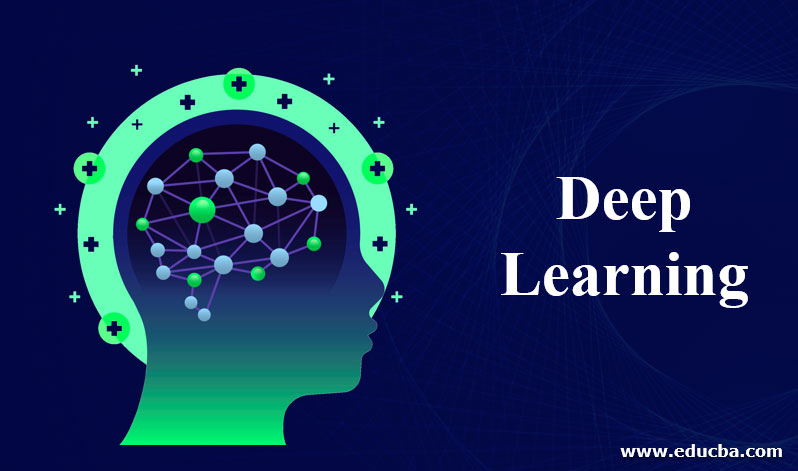SOURCEwww.educba.com

Can we make machines think like a human? Yes, we can. This thinking is the era when Deep Learning came into existence. Deep Learning is the subset of Machine learning where we develop intelligent algorithms which can mimic the human brain. Now a question that arises over here is that how do we mimic the human brain? What is the composition of the brain? Well, a brain is primarily composed of neurons that send and receive electrochemical signals through the dendrites. The processing of these signals is done inside the cell body. The output generated of these input signals is sent to the other neurons to the axon. If our task is to mimic the human brain, all we have to do is create artificial neurons, which work the same as the biological neurons. So in order to implement Deep Learning, we'll have to create an artificial neural network consisting of three layers, an input layer, a hidden layer, and the output layer.

All the inputs are taken from the input layer. Processing is done inside the hidden layer, and the output is received through the output layer.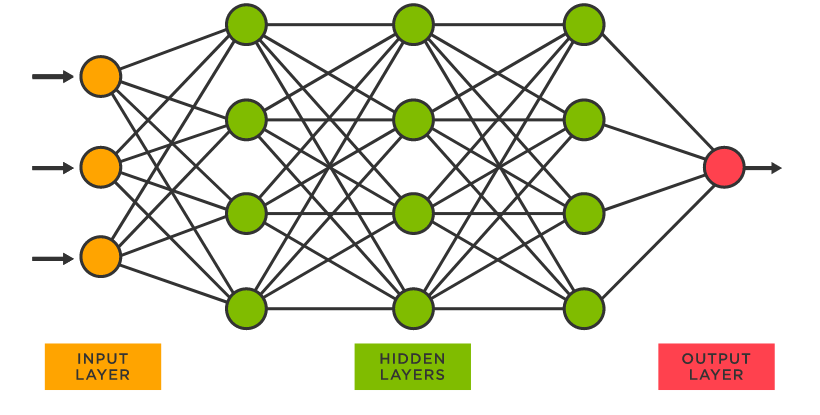SOURCEtibco.com

ARTIFICIAL NEURAL NETWORK

So, Machine learning and Deep Learning are the methods to achieve Artificial Intelligence.

### Relation Between AI, ML, DL and DS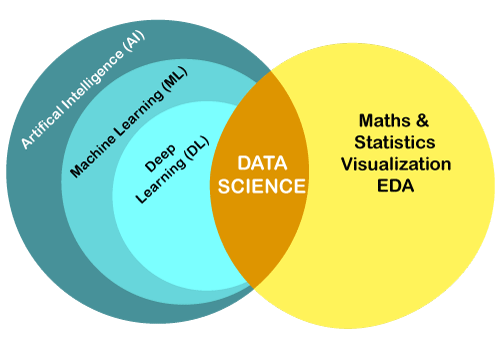SOURCE: javapoint.com

Now the question comes to where Data Science fits into this. Data Science uses the technique of machine learning and Deep Learning along with some statistical tools to structure the data to gather information about the data.

### FAQs

Q1) What is the difference between Data and Information ?

-> Data may be collected either in structured form or unstructured form. Unstructured data is very difficult to deal with. We can’t make much inference with this. So the data needs to be in structured form. The Structured form of data used to gather the inferences is called Information.

Q2) What are common ways for collecting the data ?

-> The common ways for collecting the data are as follows:

1. Surveys
2. Using APIs
3. Using Social Media Monitoring
4. Web Scraping, etc

Q3) What is Linear Regression?

-> Linear regression is an algorithm used in Supervised learning to predict the output modelling with a linear function. The algorithm tries to fit the best possible line in the datasets having minimum sum squared error with respect to each data point. We can have multivariate regression where the Gradient descent algorithm is used.

Q4) List some common Machine Learning algorithms.

-> The commonly used Machine Learning algorithm are-

1. Logistic regression
2. Linear regression
3. Random forest
4. K-means clustering
5. Cluster analysis
6. Apriori algorithm

5) What is the role of Statistics in Data Science?

-> Statistics is important for Data Science for the following reasons-

1. Helps in summarizing the data.
2. Helps in transforming raw data into information that can be understood.
3. Helps in making inferences about the whole data from the sample data.
4. Helps in predicting the future outcomes.

### Key Takeaways

So that is the end of the discussion. I hope you learned something interesting in this blog. Data Science is a very vast domain and  You may take your career ahead in this field if you find it interesting.

You could refer it from: https://www.codingninjas.com/courses/machine-learning

Thanks a lot, Happy Learning 😊